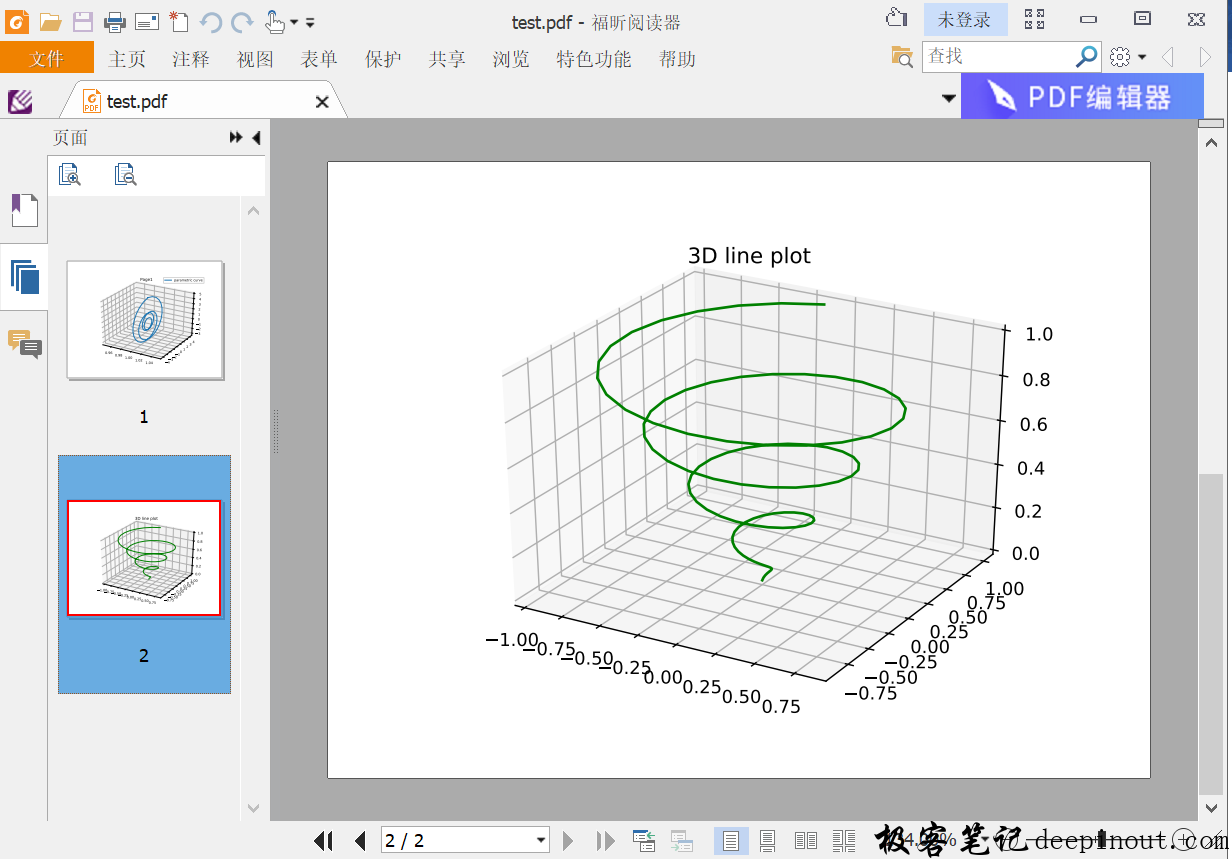# Matplotlib 保存绘图结果为PDF文件

matplotlib可以把结果以多种方式保存，比如以PNG文件，以MP4文件，以GIF文件等等，但是PDF文件在日常生活里使用也很高，因为电子书比较喜欢使用PDF的方式出版，这样可以跨不同的平台共享文件，而文件的格式不会改变，并且很容易输出纸质版本。既然PDF有这么大的应用，那么matplotlib也不会缺席这样的功能，并且它输出PDF文件的过程非常简单，只要数行代码就可以解决。

class matplotlib.backends.backend_pdf.PdfPages(filename, keep_empty=True, metadata=None)


• filename：表示保存文件的名称，或路径名称。

• keep_empty：布尔类型，当设置为False时，当PDF文件为空时自动删除，否则保留。import numpy as np
from matplotlib import pyplot as plt
from mpl_toolkits import mplot3d
from matplotlib.backends.backend_pdf import PdfPages

plt.rcParams['legend.fontsize'] = 10

#
with PdfPages('test.pdf') as pdf:
#第一页
fig = plt.figure()
ax = fig.add_subplot(1,1,1, projection = "3d")

#
theta = np.linspace(-4 * np.pi, 4 * np.pi, 100)
z = np.linspace(-2, 2, 100)
r = z**2 + 1
x = r * np.sin(theta)
y = r * np.cos(theta)

ax.plot(x, y, 1.0, label='parametric curve', zdir = 'x')
ax.legend()
plt.title('Page1')

pdf.savefig(fig)
plt.close()

#第二页
fig = plt.figure()

# 3-D投影创建
ax = plt.axes(projection ='3d')

# 定义3个坐标轴的数据
z = np.linspace(0, 1, 100)
x = z * np.sin(25 * z)
y = z * np.cos(25 * z)

# 连接所有点成曲线
ax.plot3D(x, y, z, 'green')
ax.set_title('3D line plot')

pdf.savefig(fig)
plt.close()


with PdfPages('test.pdf') as pdf


• 暂无文章

• 微信订阅